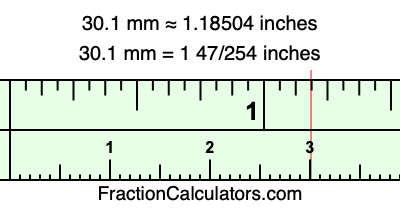30.1 mm in inchesHere is how to convert 30.1 mm to inches. We will give you the fractional answer, the decimal answer, and illustrate the answer on our tape measure.

One millimeter is equal to 5/127 inches, and 30.1 as a fraction is 301/10. Therefore, to get the fractional answer to 30.1 mm in inches, we multiply 301/10 by 5/127 and simplify if necessary.

cm × 5/127 = inches
301/10 × 5/127 = inches
301/10 × 5/127 = 1 47/254
301/10 mm = 1 47/254 inches
30.1 mm = 1 47/254 inches

As promised above, we also have the answer to 30.1 mm in inches in decimal form. Although the fractional answer above is exact, the decimal answer may be rounded if necessary:

1 47/254 ≈ 1.18503937007874
1 47/254 ≈ 1.18504
30.1 mm ≈ 1.18504 inches

Our image below shows 30.1 mm on a tape measure. The top row of the tape measure is inches, and each mark on the bottom row is 1 millimeter (10 millimeter marks equal 1 centimeter).As you can see, we drew a red line where 30.1 mm and 1.18504 inches meet on the measuring tape.

Millimeters to Inches Calculator
Here you can convert another length in millimeters to inches.

Convert  mm to inches.

What is 30.2 mm in inches?
Here is another inches to millimeters calculation that we have done for you.